# Hypothesis Testing

using computer simulation. Based on examples from the `infer` package. Code for Quiz 13.

Load the R packages we will use.

``````library(tidyverse)
library(infer)
library(skimr)
``````
• Replace all the instances of ???. These are answers on your moodle quiz.
• Run all the individual code chunks to make sure the answers in this file correspond with your quiz answers
• After you check all your code chunks run then you can knit it. It won’t knit until the ??? are replaced
• Save a plot to be your preview plot

# Question: t-test

• The data this quiz uses is a subset of `HR`
• Look at the variable definitions
• Note that the variables evaluation and salary have been recoded to be represented as words instead of numbers
• Set random seed generator to 123
``````set.seed(123)
``````

hr_1_tidy.csv is the name of your data subset

• Read it into and assign to `hr`
• Note: col_types = “fddfff” defines the column types factor-double-double-factor-factor-factor
``````hr  <- read_csv("https://estanny.com/static/week13/data/hr_1_tidy.csv",
col_types = "fddfff")
``````

use the `skim` to summarize the data in `hr`

``````skim(hr)
``````
 Name hr Number of rows 500 Number of columns 6 _______________________ Column type frequency: factor 4 numeric 2 ________________________ Group variables None

Variable type: factor

skim_variable n_missing complete_rate ordered n_unique top_counts
gender 0 1 FALSE 2 fem: 260, mal: 240
evaluation 0 1 FALSE 4 bad: 153, fai: 142, goo: 106, ver: 99
salary 0 1 FALSE 6 lev: 93, lev: 92, lev: 91, lev: 84
status 0 1 FALSE 3 fir: 185, pro: 162, ok: 153

Variable type: numeric

skim_variable n_missing complete_rate mean sd p0 p25 p50 p75 p100 hist
age 0 1 40.60 11.58 20.2 30.37 41.00 50.82 59.9 ▇▇▇▇▇
hours 0 1 49.32 13.13 35.0 37.55 45.25 58.45 79.7 ▇▂▃▂▂

The mean hours worked per week is: 49.3

## Q: Is the mean number of hours worked per week 48?

`specify` that hours is the variable of interest

``````hr  %>%
specify(response = hours)
``````
``````Response: hours (numeric)
# A tibble: 500 × 1
hours
<dbl>
1  36.5
2  55.8
3  35
4  52
5  35.1
6  36.3
7  40.1
8  42.7
9  66.6
10  35.5
# … with 490 more rows``````

hypothesize that the average hours worked is 48

``````hr  %>%
specify(response = hours)  %>%
hypothesize(null = "point", mu = 48)
``````
``````Response: hours (numeric)
Null Hypothesis: point
# A tibble: 500 × 1
hours
<dbl>
1  36.5
2  55.8
3  35
4  52
5  35.1
6  36.3
7  40.1
8  42.7
9  66.6
10  35.5
# … with 490 more rows``````

generate 1000 replicates representing the null hypothesis

``````hr %>%
specify(response = hours)  %>%
hypothesize(null = "point", mu = 48)  %>%
generate(reps = 1000, type = "bootstrap")
``````
``````Response: hours (numeric)
Null Hypothesis: point
# A tibble: 500,000 × 2
# Groups:   replicate [1,000]
replicate hours
<int> <dbl>
1         1  33.7
2         1  34.9
3         1  46.6
4         1  33.8
5         1  61.2
6         1  34.7
7         1  37.9
8         1  39.0
9         1  62.8
10         1  50.9
# … with 499,990 more rows``````

The output has 500,000 rows

calculate the distribution of statistics from the generated data

• Assign the output `null_t_distribution`
• Display `null_t_distribution`
``````null_t_distribution  <- hr  %>%
specify(response = hours)  %>%
hypothesize(null = "point", mu = 48)  %>%
generate(reps = 1000, type = "bootstrap")  %>%
calculate(stat = "t")

null_t_distribution
``````
``````Response: hours (numeric)
Null Hypothesis: point
# A tibble: 1,000 × 2
replicate   stat
<int>  <dbl>
1         1 -0.222
2         2 -0.912
3         3  1.61
4         4  0.318
5         5 -0.915
6         6 -0.538
7         7  0.307
8         8 -0.147
9         9 -0.520
10        10 -0.238
# … with 990 more rows``````
• null_t_distribution has 1000 t-stats

visualize the simulated null distribution

``````visualize(null_t_distribution)
``````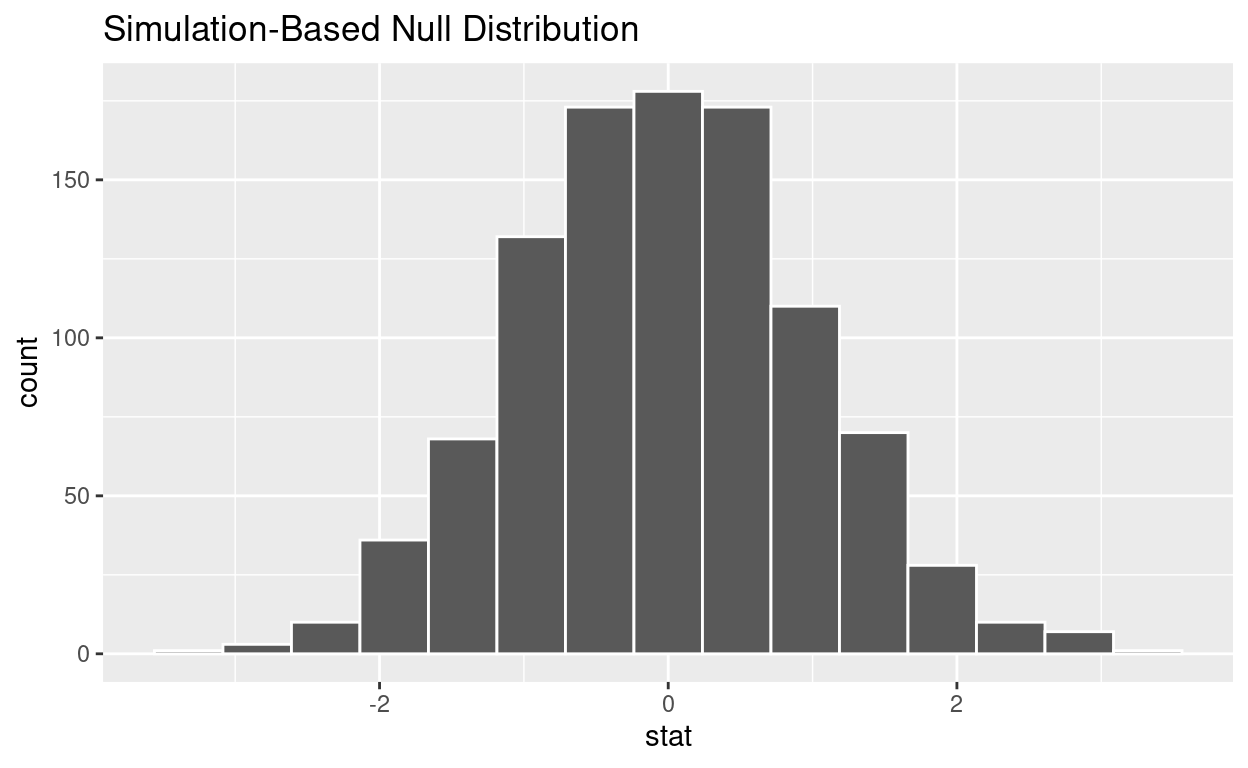calculate the statistic from your observed data * Assign the output observed_t_statistic * Display observed_t_statistic

``````observed_t_statistic  <- hr  %>%
specify(response = hours)  %>%
hypothesize(null = "point", mu = 48)  %>%
calculate(stat = "t")

observed_t_statistic
``````
``````Response: hours (numeric)
Null Hypothesis: point
# A tibble: 1 × 1
stat
<dbl>
1  2.25``````

get_p_value from the simulated null distribution and the observed statistic

``````null_t_distribution  %>%
get_p_value(obs_stat = observed_t_statistic, direction = "two-sided")
``````
``````# A tibble: 1 × 1
p_value
<dbl>
1   0.028``````

shade_p_value on the simulated null distribution

``````null_t_distribution  %>%
visualize() +
shade_p_value(obs_stat = observed_t_statistic, direction = "two-sided")
``````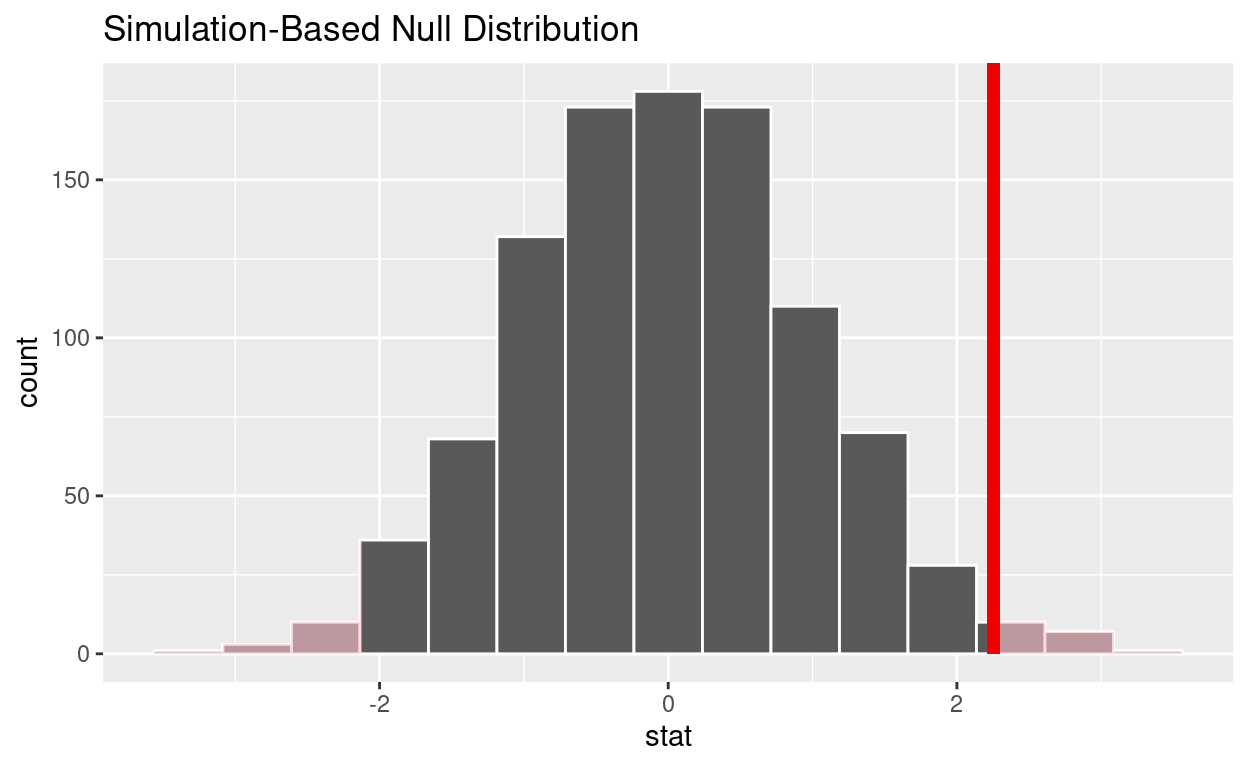Is the p-value < 0.05? yes

Does your analysis support the null hypothesis that the true mean number of hours worked was 48? no

# Question: 2 sample t-test

hr_2_tidy.csv is the name of your data subset * Read it into and assign to hr_2 * Note: col_types = “fddfff” defines the column types factor-double-double-factor-factor-factor

``````hr_2 <- read_csv("https://estanny.com/static/week13/data/hr_2_tidy.csv",
col_types = "fddfff")
``````

## Q: Is the average number of hours worked the same for both genders?

use `skim` to summarize the data in `hr_2` by gender

``````hr_2 %>%
group_by(gender)  %>%
skim()
``````
 Name Piped data Number of rows 500 Number of columns 6 _______________________ Column type frequency: factor 3 numeric 2 ________________________ Group variables gender

Variable type: factor

skim_variable gender n_missing complete_rate ordered n_unique top_counts
evaluation male 0 1 FALSE 4 bad: 79, fai: 68, goo: 61, ver: 48
evaluation female 0 1 FALSE 4 bad: 75, fai: 74, ver: 48, goo: 47
salary male 0 1 FALSE 6 lev: 49, lev: 48, lev: 48, lev: 44
salary female 0 1 FALSE 6 lev: 47, lev: 46, lev: 41, lev: 39
status male 0 1 FALSE 3 fir: 93, pro: 90, ok: 73
status female 0 1 FALSE 3 fir: 101, pro: 89, ok: 54

Variable type: numeric

skim_variable gender n_missing complete_rate mean sd p0 p25 p50 p75 p100 hist
age male 0 1 38.63 11.57 20.3 28.50 37.85 49.52 59.6 ▇▇▆▆▆
age female 0 1 41.14 11.43 20.3 31.30 41.60 50.90 59.9 ▆▅▇▇▇
hours male 0 1 49.30 13.24 35.0 37.35 46.00 59.23 79.9 ▇▃▂▂▂
hours female 0 1 49.49 13.08 35.0 37.68 45.05 58.73 78.4 ▇▃▃▂▂
• Females worked an average of `49.5` hours per week
• Males worked an average of `49.3` hours per week

Use geom_boxplot to plot distributions of hours worked by gender

``````hr_2 %>%
ggplot(aes(x = gender, y = hours)) +
geom_boxplot()
``````specify the variables of interest are hours and gender

``````hr_2 %>%
specify(response = hours, explanatory = gender)
``````
``````Response: hours (numeric)
Explanatory: gender (factor)
# A tibble: 500 × 2
hours gender
<dbl> <fct>
1  78.1 male
2  35.1 female
3  36.9 female
4  38.5 male
5  36.1 male
6  78.1 female
7  76   female
8  35.6 female
9  35.6 male
10  56.8 male
# … with 490 more rows``````

hypothesize that the number of hours worked and gender are independent

``````hr_2  %>%
specify(response = hours, explanatory = gender)  %>%
hypothesize(null = "independence")
``````
``````Response: hours (numeric)
Explanatory: gender (factor)
Null Hypothesis: independence
# A tibble: 500 × 2
hours gender
<dbl> <fct>
1  78.1 male
2  35.1 female
3  36.9 female
4  38.5 male
5  36.1 male
6  78.1 female
7  76   female
8  35.6 female
9  35.6 male
10  56.8 male
# … with 490 more rows``````

generate 1000 replicates representing the null hypothesis

``````hr_2 %>%
specify(response = hours, explanatory = gender)  %>%
hypothesize(null = "independence")  %>%
generate(reps = 1000, type = "permute")
``````
``````Response: hours (numeric)
Explanatory: gender (factor)
Null Hypothesis: independence
# A tibble: 500,000 × 3
# Groups:   replicate [1,000]
hours gender replicate
<dbl> <fct>      <int>
1  47.8 male           1
2  60.3 female         1
3  46.5 female         1
4  37.2 male           1
5  74.1 male           1
6  35.9 female         1
7  35.6 female         1
8  54.5 female         1
9  55.6 male           1
10  44.1 male           1
# … with 499,990 more rows``````

The output has 500,000 rows

calculate the distribution of statistics from the generated data

• Assign the output `null_distribution_2_sample_permute`
• Display `null_distribution_2_sample_permute`
``````null_distribution_2_sample_permute  <- hr_2 %>%
specify(response = hours, explanatory = gender)  %>%
hypothesize(null = "independence")  %>%
generate(reps = 1000, type = "permute")  %>%
calculate(stat = "t", order = c("female", "male"))

null_distribution_2_sample_permute
``````
``````Response: hours (numeric)
Explanatory: gender (factor)
Null Hypothesis: independence
# A tibble: 1,000 × 2
replicate   stat
<int>  <dbl>
1         1  0.505
2         2 -0.650
3         3  0.279
4         4  0.435
5         5  1.73
6         6 -0.139
7         7 -2.14
8         8  0.274
9         9  0.766
10        10  1.52
# … with 990 more rows``````
• `null_t_distribution` has 1000 t-stats

visualize the simulated null distribution

``````visualize(null_distribution_2_sample_permute)
``````calculate the statistic from your observed data

• Assign the output `observed_t_2_sample_stat`
• Display `observed_t_2_sample_stat`
``````observed_t_2_sample_stat  <- hr_2 %>%
specify(response = hours, explanatory = gender)  %>%
calculate(stat = "t", order = c("female", "male"))

observed_t_2_sample_stat
``````
``````Response: hours (numeric)
Explanatory: gender (factor)
# A tibble: 1 × 1
stat
<dbl>
1 0.160``````

get_p_value from the simulated null distribution and the observed statistic

``````null_t_distribution  %>%
get_p_value(obs_stat = observed_t_2_sample_stat, direction = "two-sided")
``````
``````# A tibble: 1 × 1
p_value
<dbl>
1   0.838``````

shade_p_value on the simulated null distribution

``````null_t_distribution  %>%
visualize() +
shade_p_value(obs_stat = observed_t_2_sample_stat, direction = "two-sided")
``````Is the p-value < 0.05? no

Does your analysis support the null hypothesis that the true mean number of hours worked by female and male employees was the same? yes

# Question: ANOVA

hr_1_tidy.csv is the name of your data subset * Read it into and assign to `hr_anova` * Note: col_types = “fddfff” defines the column types factor-double-double-factor-factor-factor

``````hr_anova <- read_csv("https://estanny.com/static/week13/data/hr_1_tidy.csv",
col_types = "fddfff")
``````

## Q: Is the average number of hours worked the same for all three status (fired, ok and promoted) ?

use `skim` to summarize the data in `hr_anova` by `status`

``````hr_anova %>%
group_by(status)  %>%
skim()
``````
 Name Piped data Number of rows 500 Number of columns 6 _______________________ Column type frequency: factor 3 numeric 2 ________________________ Group variables status

Variable type: factor

skim_variable status n_missing complete_rate ordered n_unique top_counts
gender fired 0 1 FALSE 2 fem: 96, mal: 89
gender ok 0 1 FALSE 2 fem: 77, mal: 76
gender promoted 0 1 FALSE 2 fem: 87, mal: 75
evaluation fired 0 1 FALSE 4 bad: 65, fai: 63, goo: 31, ver: 26
evaluation ok 0 1 FALSE 4 bad: 69, fai: 59, goo: 15, ver: 10
evaluation promoted 0 1 FALSE 4 ver: 63, goo: 60, fai: 20, bad: 19
salary fired 0 1 FALSE 6 lev: 41, lev: 37, lev: 32, lev: 32
salary ok 0 1 FALSE 6 lev: 40, lev: 37, lev: 29, lev: 23
salary promoted 0 1 FALSE 6 lev: 37, lev: 35, lev: 29, lev: 23

Variable type: numeric

skim_variable status n_missing complete_rate mean sd p0 p25 p50 p75 p100 hist
age fired 0 1 38.64 11.43 20.2 28.30 38.30 47.60 59.6 ▇▇▇▅▆
age ok 0 1 41.34 12.11 20.3 31.00 42.10 51.70 59.9 ▆▆▆▆▇
age promoted 0 1 42.13 10.98 21.0 33.40 42.95 50.98 59.9 ▆▅▆▇▇
hours fired 0 1 41.67 7.88 35.0 36.10 38.90 43.90 75.5 ▇▂▁▁▁
hours ok 0 1 48.05 11.65 35.0 37.70 45.60 56.10 78.2 ▇▃▃▂▁
hours promoted 0 1 59.27 12.90 35.0 51.12 60.10 70.15 79.7 ▆▅▇▇▇
• Employees that were `fired` worked an average of `41.7` hours per week
• Employees that were `ok` worked an average of `48.0` hours per week
• Employees that were `promoted` worked an average of `59.3` hours per week

Use `geom_boxplot` to plot distributions of hours worked by status

``````hr_anova %>%
ggplot(aes(x = status, y = hours)) +
geom_boxplot()
``````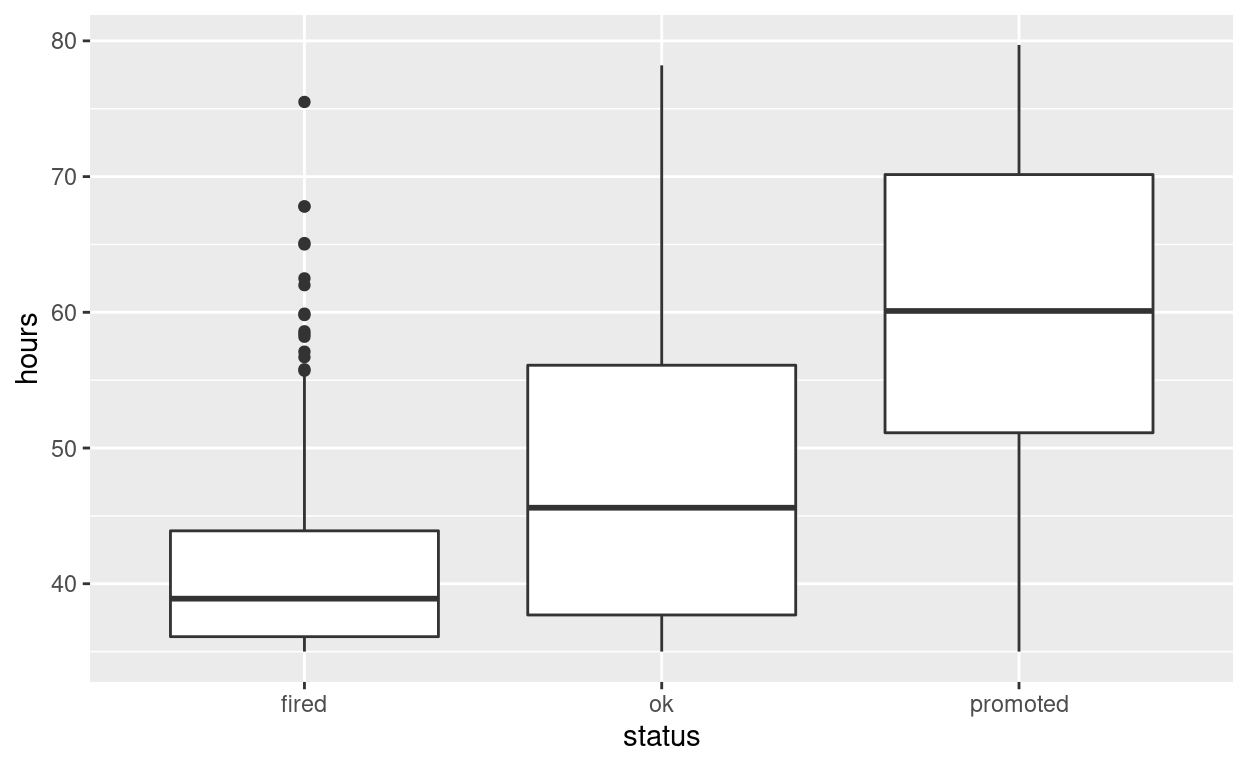`specify` the variables of interest are `hours` and `status`

``````hr_anova %>%
specify(response = hours, explanatory = status)
``````
``````Response: hours (numeric)
Explanatory: status (factor)
# A tibble: 500 × 2
hours status
<dbl> <fct>
1  36.5 fired
2  55.8 ok
3  35   fired
4  52   promoted
5  35.1 ok
6  36.3 ok
7  40.1 promoted
8  42.7 fired
9  66.6 promoted
10  35.5 ok
# … with 490 more rows``````

`hypothesize` that the number of hours worked and status are independent

``````hr_anova  %>%
specify(response = hours, explanatory = status)  %>%
hypothesize(null = "independence")
``````
``````Response: hours (numeric)
Explanatory: status (factor)
Null Hypothesis: independence
# A tibble: 500 × 2
hours status
<dbl> <fct>
1  36.5 fired
2  55.8 ok
3  35   fired
4  52   promoted
5  35.1 ok
6  36.3 ok
7  40.1 promoted
8  42.7 fired
9  66.6 promoted
10  35.5 ok
# … with 490 more rows``````

`generate` 1000 replicates representing the null hypothesis

``````hr_anova %>%
specify(response = hours, explanatory = status)  %>%
hypothesize(null = "independence")  %>%
generate(reps = 1000, type = "permute")
``````
``````Response: hours (numeric)
Explanatory: status (factor)
Null Hypothesis: independence
# A tibble: 500,000 × 3
# Groups:   replicate [1,000]
hours status   replicate
<dbl> <fct>        <int>
1  40.3 fired            1
2  40.3 ok               1
3  37.3 fired            1
4  50.5 promoted         1
5  35.1 ok               1
6  67.8 ok               1
7  39.3 promoted         1
8  35.7 fired            1
9  40.2 promoted         1
10  38.4 ok               1
# … with 499,990 more rows``````

The output has 500,000 rows

calculate the distribution of statistics from the generated data

• Assign the output `null_distribution_anova`
• Display `null_distribution_anova`
``````null_distribution_anova  <- hr_anova %>%
specify(response = hours, explanatory = status)  %>%
hypothesize(null = "independence")  %>%
generate(reps = 1000, type = "permute")  %>%
calculate(stat = "F")

null_distribution_anova
``````
``````Response: hours (numeric)
Explanatory: status (factor)
Null Hypothesis: independence
# A tibble: 1,000 × 2
replicate   stat
<int>  <dbl>
1         1 0.667
2         2 2.78
3         3 1.24
4         4 0.330
5         5 2.08
6         6 1.95
7         7 0.243
8         8 0.312
9         9 0.440
10        10 0.0281
# … with 990 more rows``````
• null_distribution_anova has 1000 F-stats

`visualize` the simulated null distribution

``````visualize(null_distribution_anova)
``````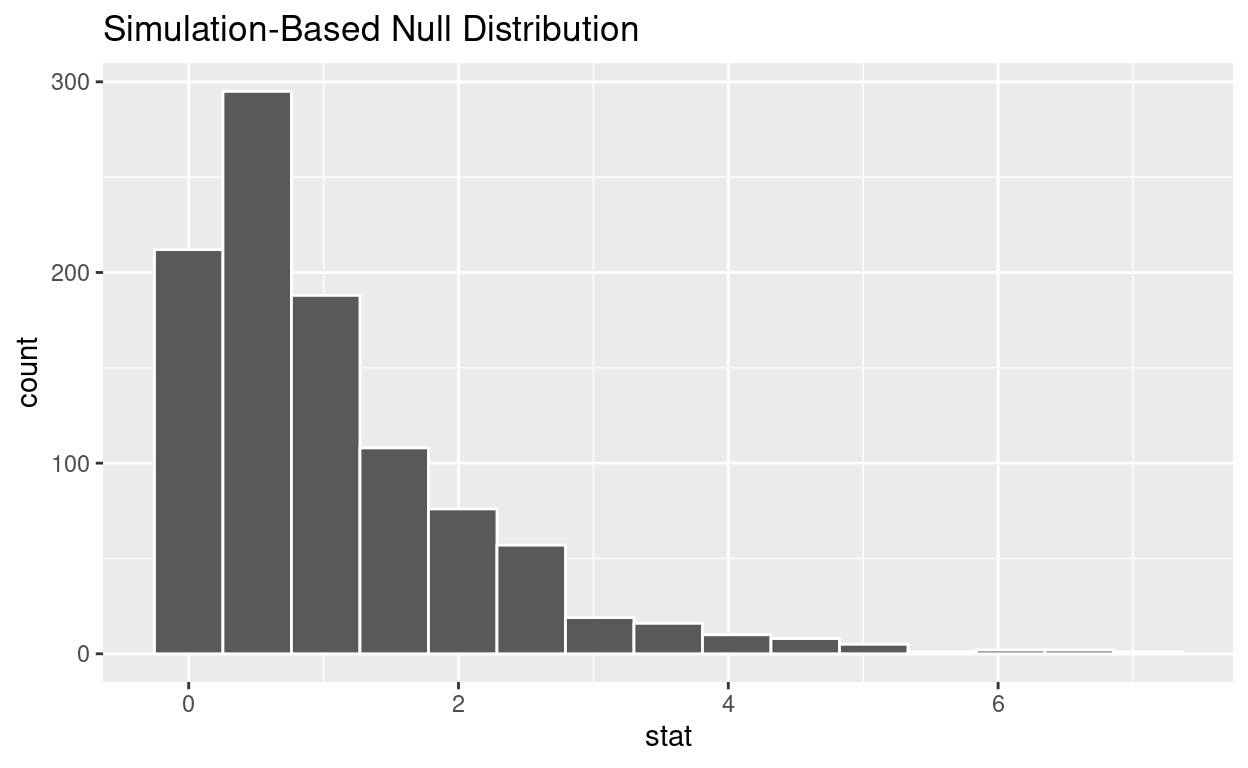`calculate` the statistic from your observed data * Assign the output `observed_f_sample_stat` * Display `observed_f_sample_stat`

``````observed_f_sample_stat  <- hr_anova %>%
specify(response = hours, explanatory = status)  %>%
calculate(stat = "F")

observed_f_sample_stat
``````
``````Response: hours (numeric)
Explanatory: status (factor)
# A tibble: 1 × 1
stat
<dbl>
1  115.``````

get_p_value from the simulated null distribution and the observed statistic

``````null_distribution_anova  %>%
get_p_value(obs_stat = observed_f_sample_stat, direction = "greater")
``````
``````# A tibble: 1 × 1
p_value
<dbl>
1       0``````

`shade_p_value` on the simulated null distribution

``````null_t_distribution  %>%
visualize() +
shade_p_value(obs_stat = observed_f_sample_stat, direction = "greater")
``````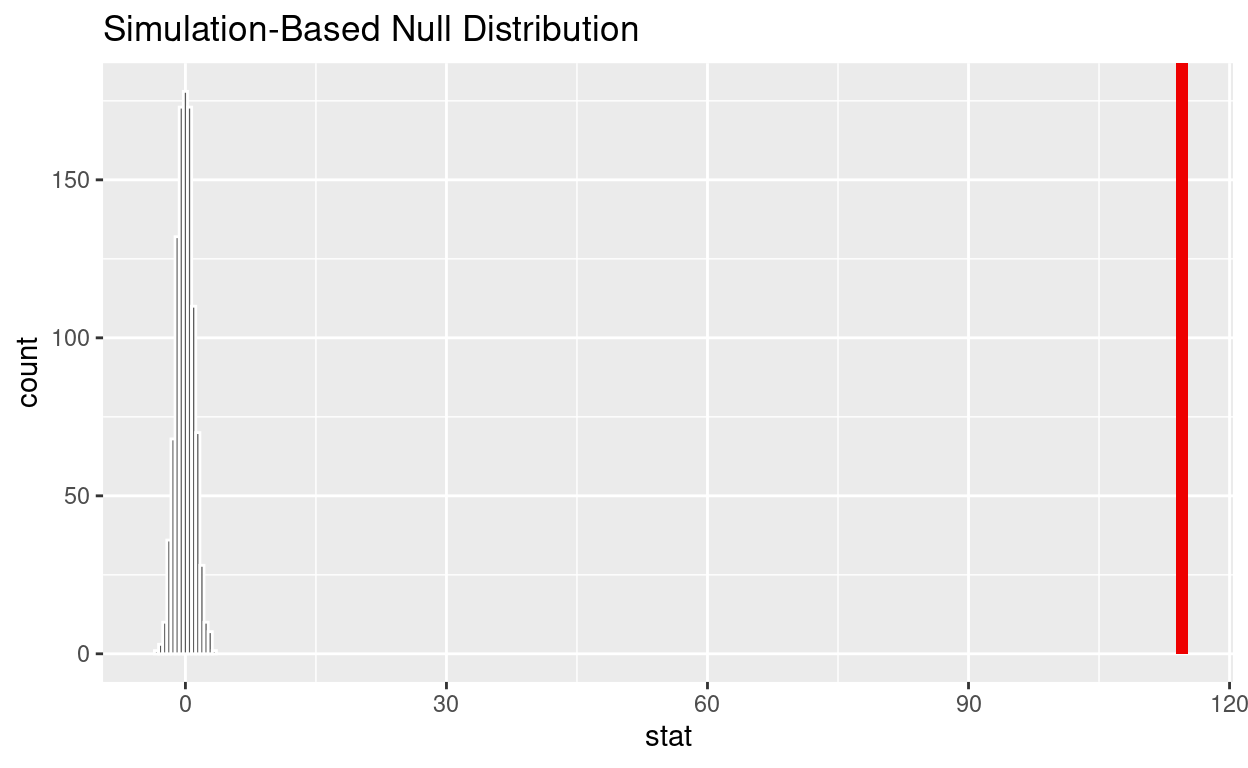Save the previous plot to preview.png and add to the yaml chunk at the top

``````ggsave(filename = "preview.png",
path = here::here("_posts", "2022-05-03-hypothesis-testing"))
``````

If the p-value < 0.05? yes

Does your analysis support the null hypothesis that the true means of the number of hours worked for those that were “fired”, “ok” and “promoted” were the same? no